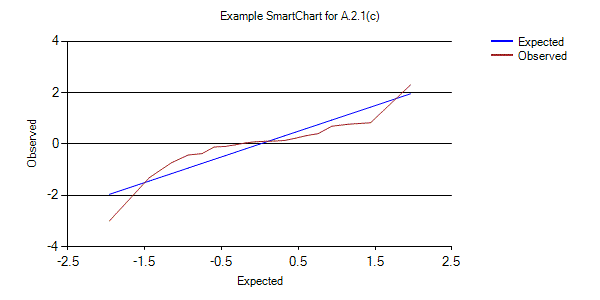/

### Extreme Events – Specimen Question A.2.1(c) – Answer/Hints

Q. Prepare a standardised quantile-quantile plot for Index A. Does it appear to be fat-tailed?

A standardised quantile-quantile plot for Index A can be derived using the following steps:

i.            Standardise the data, i.e. if the original logged returns are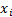then calculate: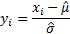where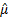is the sample mean and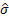is the sample standard deviation

ii.            Order this data, i.e. calculate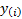where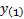is the smallest value of the set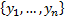,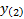is the next smallest etc.

iii.            Calculate the ‘expected’ value that we would expect each of theto take were they to be coming from a normal distribution, i.e.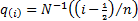iv.            Plot theagainst the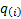, usually with thealong the horizontal axis and thealong the vertical axis.

Steps (i) to (iii) can be all be carried out without too much effort on a case-by-case basis in a spreadsheet system such as Microsoft Excel or using the Nematrian web functions MnNormaliseArray (to carry out steps (i) and (ii) and MnStandardisedNormalQuantilesArray (to carry out step (iii)).

However, it is simpler to use the Nematrian website charting capability. This includes a web function, i.e. MnPlotStandardisedQQ, which both creates (internally) the required data to be viewed in a standardised QQ-plot (i.e. carries out steps (i) to (iii) above) and also creates a temporary Nematrian ‘SmartChart’, i.e. the plot itself as in step (iv), accessible via a suitable ‘SmartCode’. As with other Nematrian web functions it is possible to access the function programmatically (in which case it merely returns the temporary ‘SmartCode’ assigned to the relevant ‘SmartChart’). It can also be accessed interactively, in which case the output on the webpage includes both the ‘SmartCode’ and the SmartChart.

For users with appropriate permissions, temporary SmartCharts can be converted into permanent SmartCharts, which are stored permanently on the Nematrian website and retain an association between the creator of the chart, its underlying data and its visual form. These can be copied and pasted from the Nematrian website into other venues, e.g. Word documents or Powerpoint presentations. For users without these permissions the image forming the SmartChart can be copied in a manner similar to any other image you might find on a webpage, but what is copied no longer retains an association with its creator and its underlying data.

A permanent SmartChart answering this question is: